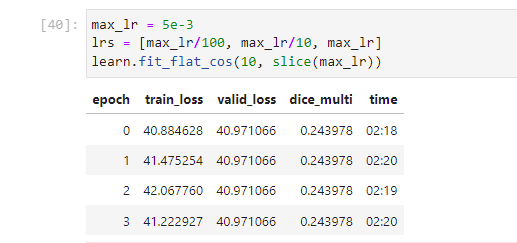# GI Tract Image Segmentation kaggle

Hi ,

I have been trying to use fastai for the latest image segmentation competition on kaggle (UW-Madison GI Tract Image Segmentation | Kaggle). I tried to implement unet in fastai using unet learner but the problem is that dice metric stays constant at every epoch , i.e it is basically predicting every pixel to be 0 instead 0f [0,1,2,3]. The notebook can be viewed here - gi-tract/fastai_unet.ipynb at main · msadiva/gi-tract · GitHub .I think the right way should be:
`max_lr = 5e-3`
`lrs = slice(max_lr /100 max_lr /10)`

1. you might need to unfreeze it.
`learn.unfreeze()`
2. You use max_lr not the sliced one. Maybe this works.
`learn.fit_flat_cos(10, lrs)`

I have tried different lrs but result is always the same, DiceMulti remains constant at every epoch

I remember having this problem with the Dice metric sometime ago too. I can’t seem to find my colab notebook that I was experimenting with, but if I can remember correctly, the `axis` parameter helped me fix constant Dice metrics (When initializing the metric)

I’ll post an update when I find the Colab Notebook, but in the meantime you can try and see if that solves it for you.

UPDATE:
I was going through your notebook and I noticed you passed in metrics like this `metrics=DiceMulti`
try `metrics=DiceMulti()`

1 Like